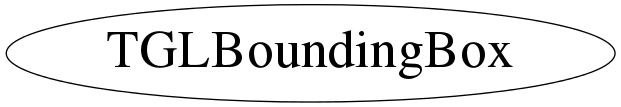# class TGLBoundingBox

```
TGLBoundingBox

Concrete class describing an orientated (free) or axis aligned box
of 8 verticies. Supports methods for setting aligned or orientated
boxes, find volume, axes, extents, centers, face planes etc.
Also tests for overlap testing of planes and other bounding boxes,
with fast sphere approximation.

```

## Function Members (Methods)

public:
 TGLBoundingBox() TGLBoundingBox(const TGLVertex3* vertex) TGLBoundingBox(const Double_t** vertex) TGLBoundingBox(const TGLBoundingBox& other) TGLBoundingBox(const TGLVertex3& lowVertex, const TGLVertex3& highVertex) virtual ~TGLBoundingBox() const TGLVector3& Axis(UInt_t i, Bool_t normalised = kTRUE) const TGLVertex3 Center() const static TClass* Class() Double_t Diagonal() const void Draw(Bool_t solid = kFALSE) const void Dump() const void ExpandAligned(const TGLVertex3& point) TGLVector3 Extents() const const vector& FaceVertices(TGLBoundingBox::EFace face) const TGLPlane GetNearPlane() const virtual TClass* IsA() const Bool_t IsEmpty() const TGLVertex3 MaxAAVertex() const void MergeAligned(const TGLBoundingBox& other) TGLVertex3 MinAAVertex() const Int_t NumVertices() const TGLBoundingBox& operator=(const TGLBoundingBox& other) const TGLVertex3& operator[](UInt_t index) const Rgl::EOverlap Overlap(const TGLPlane& plane) const Rgl::EOverlap Overlap(const TGLBoundingBox& box) const void PlaneSet(TGLPlaneSet_t& planeSet) const void Scale(Double_t factor) void Scale(Double_t xFactor, Double_t yFactor, Double_t zFactor) void Set(const TGLVertex3* vertex) void Set(const Double_t** vertex) void Set(const TGLBoundingBox& other) void SetAligned(const TGLVertex3& lowVertex, const TGLVertex3& highVertex) void SetAligned(UInt_t nbPnts, const Double_t* pnts) void SetEmpty() virtual void ShowMembers(TMemberInspector&) virtual void Streamer(TBuffer&) void StreamerNVirtual(TBuffer& ClassDef_StreamerNVirtual_b) void Transform(const TGLMatrix& matrix) void Translate(const TGLVector3& offset) const TGLVertex3& Vertex(UInt_t index) const const TGLVertex3* Vertices() const Double_t Volume() const Double_t XMax() const Double_t XMin() const Double_t YMax() const Double_t YMin() const Double_t ZMax() const Double_t ZMin() const
private:
 Double_t Max(UInt_t index) const Double_t Min(UInt_t index) const void UpdateCache() Bool_t ValidIndex(UInt_t index) const

## Data Members

public:
 enum EFace { kFaceLowX kFaceHighX kFaceLowY kFaceHighY kFaceLowZ kFaceHighZ kFaceCount };
private:
 TGLVector3 fAxes ! box axes in global frame - cached for speed TGLVector3 fAxesNorm ! normalised box axes in global frame - cached for speed Double_t fDiagonal ! max box diagonal - cached for speed TGLVertex3 fVertex ! the 8 bounding box vertices Double_t fVolume ! box volume - cached for speed

## Class Charts## Function documentation

``` Construct an empty bounding box
```
TGLBoundingBox(const TGLVertex3 vertex)
``` Construct a bounding box from provided 8 vertices
```
TGLBoundingBox(const Double_t vertex)
``` Construct a bounding box from provided 8 vertices
```
TGLBoundingBox(const TGLVertex3& lowVertex, const TGLVertex3& highVertex)
``` Construct an global axis ALIGNED bounding box from provided low/high vertex pair
```
TGLBoundingBox(const TGLBoundingBox& other)
``` Construct a bounding box as copy of existing one
```

``` Destroy bounding box
```
void UpdateCache()
``` Update the internally cached volume and axes vectors - these are retained
for efficiency - many more reads than modifications
```
void Set(const TGLVertex3 vertex)
``` Set a bounding box from provided 8 vertices
```
void Set(const Double_t vertex)
``` Set a bounding box from provided 8 vertices
```
void Set(const TGLBoundingBox& other)
``` Set a bounding box from vertices of other
```
void SetEmpty()
``` Set bounding box empty - all vertices at (0,0,0)
```
void SetAligned(const TGLVertex3& lowVertex, const TGLVertex3& highVertex)
``` Set ALIGNED box from two low/high vertices. Box axes are aligned with
global frame axes that vertices are specified in.
```
void SetAligned(UInt_t nbPnts, const Double_t* pnts)
``` Set ALIGNED box from one or more points. Box axes are aligned with
global frame axes that points are specified in.
```
void MergeAligned(const TGLBoundingBox& other)
``` Expand current bbox so that it includes other's bbox.
This make the bbox axis-aligned.
```
void ExpandAligned(const TGLVertex3& point)
``` Expand current bbox so that it includes the point.
This make the bbox axis-aligned.
```
void Scale(Double_t factor)
``` Isotropically scale bounding box along it's LOCAL axes, preserving center
```
void Scale(Double_t xFactor, Double_t yFactor, Double_t zFactor)
``` Asymetrically scale box along it's LOCAL x,y,z axes, preserving center
```
void Translate(const TGLVector3& offset)
``` Translate all vertices by offset
```
void Transform(const TGLMatrix& matrix)
``` Transform all vertices with matrix.
```
const std::vector<UInt_t> & FaceVertices(TGLBoundingBox::EFace face) const
```return a vector of face vertices
y
|
|
|________x
/  3-------2
/  /|      /|
z  7-------6 |
| 0-----|-1
|/      |
4-------5

```
void PlaneSet(TGLPlaneSet_t& planeSet) const
``` Fill out supplied plane set vector with TGLPlane objects
representing six faces of box
```
TGLPlane GetNearPlane() const
``` Return the near-plane.
```
Rgl::EOverlap Overlap(const TGLPlane& plane) const
``` Find overlap (Inside, Outside, Partial) of plane c.f. bounding box.
```
Rgl::EOverlap Overlap(const TGLBoundingBox& box) const
``` Find overlap (Inside, Outside, Partial) of other bounding box c.f. us.
```
void Draw(Bool_t solid = kFALSE) const
``` Draw the bounding box as either wireframe (default) of solid
using current GL color.
```
Double_t Min(UInt_t index) const
``` Find minimum vertex value for axis of index X(0), Y(1), Z(2)
```
Double_t Max(UInt_t index) const
``` Find maximum vertex value for axis of index X(0), Y(1), Z(2)
```
TGLVertex3 MinAAVertex() const
``` Find minimum vertex values.
```
TGLVertex3 MaxAAVertex() const
``` Find maximum vertex values.
```
void Dump() const
``` Output to std::cout the vertices, center and volume of box
```
const TGLVertex3 & Vertex(UInt_t index) const
const TGLVertex3* Vertices() const
TGLVector3 Extents() const
TGLVertex3 Center() const
const TGLVector3 & Axis(UInt_t i, Bool_t normalised = kTRUE) const
Bool_t IsEmpty() const
Bool_t ValidIndex(UInt_t index) const
`{ return (index < 8); }`
Double_t XMin() const
`{ return Min(0); }`
Double_t XMax() const
`{ return Max(0); }`
Double_t YMin() const
`{ return Min(1); }`
Double_t YMax() const
`{ return Max(1); }`
Double_t ZMin() const
`{ return Min(2); }`
Double_t ZMax() const
`{ return Max(2); }`
Int_t NumVertices() const
`{ return 8; }`
Double_t Volume() const
`{ return fVolume; }`
Double_t Diagonal() const
`{ return fDiagonal; }`